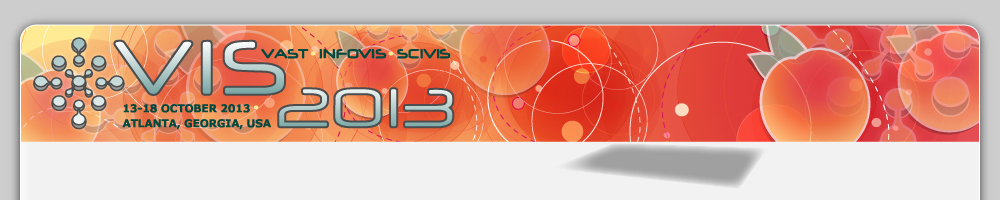13 - 18 OCTOBER 2013, ATLANTA, GEORGIA, USASelecting the Aspect Ratio of a Scatter Plot Based on Its Delaunay Triangulation

Authors:
Martin Fink, Jan-Henrik Haunert, Joachim Spoerhase, Alexander Wolff
Abstract:

Scatter plots are diagrams that visualize two-dimensional data as sets of points in the plane. They allow users to detect correlations and clusters in the data. Whether or not a user can accomplish these tasks highly depends on the aspect ratio selected for the plot, i.e., the ratio between the horizontal and the vertical extent of the diagram. We argue that an aspect ratio is good if the Delaunay triangulation of the scatter plot at this aspect ratio has some nice geometric property, e.g., a large minimum angle or a small total edge length. More precisely, we consider the following optimization problem. Given a set Q of points in the plane, find a scale factor s such that scaling the x-coordinates of the points in Q by s and the y-coordinates by 1=s yields a point set P(s) that optimizes a property of the Delaunay triangulation of P(s), over all choices of s. We present an algorithm that solves this problem efficiently and demonstrate its usefulness on real-world instances. Moreover, we discuss an empirical test in which we asked 64 participants to choose the aspect ratios of 18 scatter plots. We tested six different quality measures that our algorithm can optimize. In conclusion, minimizing the total edge length and minimizing what we call the ﾒuncompactnessﾓ of the triangles of the Delaunay triangulation yielded the aspect ratios that were most similar to those chosen by the participants in the test.